NEET  >  Test: Fluid Mechanics - 2

# Test: Fluid Mechanics - 2

Test Description

## 25 Questions MCQ Test Physics Class 11 | Test: Fluid Mechanics - 2

Test: Fluid Mechanics - 2 for NEET 2023 is part of Physics Class 11 preparation. The Test: Fluid Mechanics - 2 questions and answers have been prepared according to the NEET exam syllabus.The Test: Fluid Mechanics - 2 MCQs are made for NEET 2023 Exam. Find important definitions, questions, notes, meanings, examples, exercises, MCQs and online tests for Test: Fluid Mechanics - 2 below.
Solutions of Test: Fluid Mechanics - 2 questions in English are available as part of our Physics Class 11 for NEET & Test: Fluid Mechanics - 2 solutions in Hindi for Physics Class 11 course. Download more important topics, notes, lectures and mock test series for NEET Exam by signing up for free. Attempt Test: Fluid Mechanics - 2 | 25 questions in 50 minutes | Mock test for NEET preparation | Free important questions MCQ to study Physics Class 11 for NEET Exam | Download free PDF with solutions
 1 Crore+ students have signed up on EduRev. Have you?
Test: Fluid Mechanics - 2 - Question 1

### Some liquid is filled in a cylindrical vessel of radius R. Let F1 be the force applied by the liquid on the bottom of the cylinder. Now the same liquid is poured into a vessel of uniform square cross-section of side R. Let F2 be the force applied by the liquid on the bottom of this new vessel. Then :

Detailed Solution for Test: Fluid Mechanics - 2 - Question 1

Force applied on the base is equal to the weight of the liquid, since in both cases the same liquid has been poured in the container, it will exert the same force on the base of the container.
F1​=F2

Test: Fluid Mechanics - 2 - Question 2

### In the figure shown, the heavy cylinder (radius R) resting on a smooth surface separates two liquids of densities 2r and 3r. The height `h' for the equilibrium of cylinder must be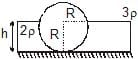Detailed Solution for Test: Fluid Mechanics - 2 - Question 2

First, let’s concentrate on the force exerted by the liquid of density 3ρ on the cylinder in the horizontal direction.

Let the length of the cylinder be L.
Consider a small segment of length rdθ at an angle θ from the horizontal line.
Height of this segment from the topmost point of fluid 3ρ is R sinθ
Hence, the pressure exerted by the fluid will be 3ρgRsinθ
The force exerted in the horizontal direction, dF=3ρgRsinθRLcosθdθ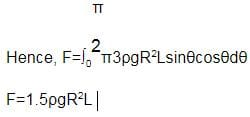Similarly, proceeding for the fluid with density 2ρ
Height of any segment, above horizontal =h−R−Rsinθ
below horizontal, h−R+Rsinθ
Thus, horizontal force on the cylinder because of fluid,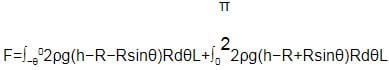For equilibrium, both the forces should be equal, hence solving the above equation,
h = R √3/2​​

Test: Fluid Mechanics - 2 - Question 3

### The vertical limbs of a U shaped tube are filled with a liquid of density r upto a height h on each side. The horizontal portion of the U tube having length 2h contains a liquid of density 2r. The U tube is moved horizontally with an accelerator g/2 parallel to the horizontal arm. The difference in heights in liquid levels in the two vertical limbs, at steady state will be

Detailed Solution for Test: Fluid Mechanics - 2 - Question 3

Given:
a=g/2​
Pressure at A
PA​=Po​+ρgh+(2ρ)g(h−x)=Po​+3ρgh−2ρgx
Pressure at B
PB​=Po​+ρgx
Using
PA​−PB​=[2ρ(h+x)+ρ(h−x)]a
∴ (Po​+3ρgh−2ρgx)−(Po​+ρgx)=[3ρh+ρx]×g/2​
OR 3ρgh−3ρgx=3​ρgh/2+1​ρgx/2
OR 3​ρgh/2=7​ρgx/2  ⟹x=3​h/7
∴ Difference in the heights between two columns ΔH=(2h−x)−x=2h−2x
⟹ ΔH=2h−6h/7​=8h/7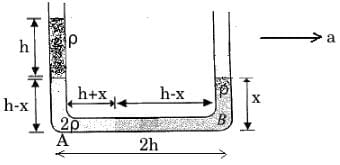Test: Fluid Mechanics - 2 - Question 4

An open cubical tank was initially fully filled with water. When the tank was accelerated on a horizontal plane along one of its side it was found that one third of volume of water spilled out. The acceleration was

Detailed Solution for Test: Fluid Mechanics - 2 - Question 4

Let say the tank is accelerating by some acceleration a, such that the rest water in the tanks forms shape like this -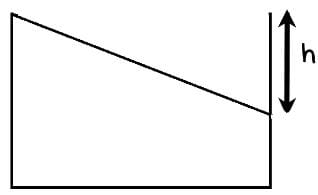Acceleration, a is right wards.
Where let say h is the height from top till which there is no water
Now if we say V is total volume and B is area of its base and S be its height
We have ½ h X B = V / 3
Thus we get h = S/3
Thus the angle in this cross section of vacant triangle is tan-1
Also the same triangle relates a and g, which can be seen when we make the block a inertial frame by adding pseudo force of magnitude ma and directing leftwards, thus we get a/g = ⅓
Thus we get a = g/3

Test: Fluid Mechanics - 2 - Question 5

A cone of radius R and height H, is hanging inside a liquid of density r by means of a string as shown in the figure. The force, due to the liquid acting on the slant surface of the cone is (neglect atmospheric pressure)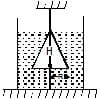Test: Fluid Mechanics - 2 - Question 6

A heavy hollow cone of radius R and height h is placed on a horizontal table surface, with its flat base on the table. The whole volume inside the cone is filled with water of density r. The circular rim of the cone's base has a watertight seal with the table's surface and the top apex of the cone has a small hole. Neglecting atmospheric pressure find the total upward force exerted by water on the cone is

Detailed Solution for Test: Fluid Mechanics - 2 - Question 6

Consider the equilibrium of water,
Weight of water(downwards) + Force due to table(upwards) + Force due to side walls(downwards)= 0
Weight of water = (1​πR2h/3)ρg
Force due to table on water = Pressure at the bottom× Area
= ρgh×πR2
Total upward force exerted by water on the cone = (ρghπR2)- (1​πR2hρg/3)
= (2/3​)πR2hρg

Test: Fluid Mechanics - 2 - Question 7

A slender homogeneous rod of length 2L floats partly immersed in water, being supported by a string fastened to one of its ends, as shown. The specific gravity of the rod is 0.75. The length of rod that extends out of water is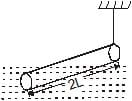Detailed Solution for Test: Fluid Mechanics - 2 - Question 7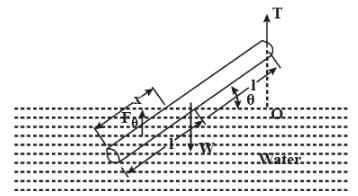Let's say x length of the rod is dipped into the water.
Since the buoyant force acts through the centre of gravity the displaced water , the condition for rotational equilibrium is, taking moments about a point O along the line of action of T,
0=Στo
⇒0=wl cosθ−FB​(2l−x/2​)cosθ
⇒0=ρrod​gA(2l)(lcosθ)−ρwater​gAx(2l−x/2​)cosθ
⇒0=(1/2​ρwater​gAcosθ) (x2−4lx+4 (ρrodwater)​l2) where A=cross section area
⇒x2−4lx+3l2=0
⇒x=l,3l.
x=3l is not a possible solution, hence 2l−x=2l−l=l length of the rod extends out of the water.

Test: Fluid Mechanics - 2 - Question 8

A dumbbell is placed in water of density r. It is observed that by attaching a mass m to the rod, the dumbbell floats with the rod horizontal on the surface of water and each sphere exactly half submerged as shown in the figure. The volume of the mass m is negligible. The value of length l is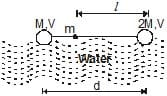Test: Fluid Mechanics - 2 - Question 9

A container of large surface area is filled with liquid of density r. A cubical block of side edge a and mass M is floating in it with four-fifth of its volume submerged. If a coin of mass m is placed gently on the top surface of the block is just submerged. M is

Test: Fluid Mechanics - 2 - Question 10

A cork of density 0.5 gcm-3 floats on a calm swimming pool. The fraction of the cork's volume which is under water is

Test: Fluid Mechanics - 2 - Question 11

Two cyllinders of same cross-section and length L but made of two material of densities d1and d2 are cemented together to form a cylinder of length 2L. The combination floats in a liquid of density d with a length L/2 above the surface of the liquid. If d1> d2 then :

Detailed Solution for Test: Fluid Mechanics - 2 - Question 11

If we make the fbd of the body we get,
(A x L x d2 + A x L x d2) g = (A x 3L/2 X d)g
Thus we get that
d1 + d2 = 3/2d
And as d2 < d1
We get d1 + d2  <  d1 + d1
Thus we get 2 d1 > 3d/2
Thus we get d1 > 3d/4

Test: Fluid Mechanics - 2 - Question 12

A small wooden ball of density r is immersed in water of density s to depth h and then released. The height H above the surface of water up to which the ball will jump out of water is

Test: Fluid Mechanics - 2 - Question 13

A hollow sphere of mass M and radius r is immersed in a tank of water (density rw). The sphere would float if it were set free. The sphere is tied to the bottom of the tank by two wires which makes angle 45º with the horizontal as shown in the figure. The tension T1 in the wire is :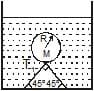Test: Fluid Mechanics - 2 - Question 14

A body having volume V and density r is attached to the bottom of a container as shown. Density of the liquid is d(>r). Container has a constant upward acceleration a. Tension in the string is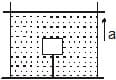Test: Fluid Mechanics - 2 - Question 15

A hollow cone floats with its axis vertical upto one-third of its height in a liquid of relative density 0.8 and with its vertex submerged. When another liquid of relative density r is filled in it upto one-third of its height, the cone floats upto half its vertical height. The height of the cone is 0.10 m and radius of the circular base is 0.05 m. The specific gravity r is given by

Detailed Solution for Test: Fluid Mechanics - 2 - Question 15

Let the volume of cylinder be V and density to be ρ1
1​g=V×800×g/27​..(1) ,Volume of cone with height is h/3 is V/27
1​g+Vρg/27=V×800g/8​ ..(2) ,Volume of cone with height is h/2 is V/8
from above equation we ρ=800×19​/8
Specific gravity =1900/1000​=1.9

Test: Fluid Mechanics - 2 - Question 16

There is a metal cube inside a block of ice which is floating on the surface of water. The ice melts completely and metal falls in the water. Water level in the

container

Detailed Solution for Test: Fluid Mechanics - 2 - Question 16

When an ice cube with an iron ball inside floating on water melts, the level of water will decrease.
To understand why this is so let us consider the action of the ice and the ball separately.
The ice floats on water because it has lower density than water. When ice is put in water it displaces a volume of water which weighs the same as the weight of ice. After melting, the volume of ice becomes the same as that of the water that it was displacing. Therefore, there is no change in level of water due to melting of ice.
Now let us examine the effect of the iron ball. The combined density of ice and iron ice is still less than that of water. That is why the ice cube with the ball floats. In this case, additional volume of water displaced due to the weight of the metal piece, weighs the same as the weight of the ball. This is more than the volume of the ball which has higher density than water. When the ice melts, the iron ball no longer is able to float above water and sinks. At this time the volume of water displaced by the metal piece that has sunk is equal to just the volume of the ball. Thus the volume of water displaced by the ball reduces on melting of ice. Therefore, the level of water will reduce after melting of ice.
Please note that, if at any time, before complete melting of ice, the ball embedded in ice sinks in the water, temporarily the level of water will rise. The exact amount of rise will depend on the remaining quantity of ice, If only the ball sinks, but the ice cubes remains floating, then also the water level will reduce to the same level as after complete melting of ice.

Test: Fluid Mechanics - 2 - Question 17

A uniform solid cylinder of density 0.8 g/cm3 floats in equilibrium in a combination of two non-mixing liquid A and B with its axis vertical. The densities of liquid A and B are 0.7 g/cm3 and 1.2 gm/cm3. The height of liquid A is hA = 1.2 cm and the length of the part of cylinder immersed in liquid B is hB = 0.8 cm. Then the length of the cylinder in air is

Test: Fluid Mechanics - 2 - Question 18

A cylindrical tank of height 1 m and cross section area A = 4000 cm2 is initially empty when it is kept under a tap of cross sectional area 1 cm2. Water starts flowing from the tap at t = 0, with a speed = 2 m/s. There is a small hole in the base of the tank of cross-sectional area 0.5 cm2. The variation of height of water in tank (in meters) with time t is best depicted by

Detailed Solution for Test: Fluid Mechanics - 2 - Question 18

We know the velocity of the water from the tap and the cross-sectional area of the tank. There is a rise in water level and as the water level rises the velocity of efflux through the bottom hole also increases.

Test: Fluid Mechanics - 2 - Question 19

A cubical box of wine has a small spout located in one of the bottom corners. When the box is full and placed on a level surface, opening the spout results in a flow of wine with a initial speed of v0 (see figure). When the box is half empty, someone tilts it at 45º so that the spout is at the lowest point (see figure). When the spout is opened the wine will flow out with a speed of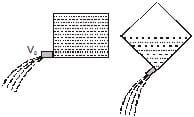Detailed Solution for Test: Fluid Mechanics - 2 - Question 19

According to Torricelli's Theorem velocity of efflux i.e. the velocity with which the liquid flows out of a hole is equal to √2gh​ where h is the depth of the hole below the liquid surface.
Let’s say side of the cube is a, so we have
vo​=√2ga​
When cubical box is half empty, height of wine surface above the spout is half of the diagonal of the cube's face, i.e. (√2​a)/2​=​a​/√2
Now the speed of the wine from the spout is v′=√2g(a​/√2​)​=vo/(2)1/4

Test: Fluid Mechanics - 2 - Question 20

A vertical cylindrical container of base area A and upper cross-section area A1 making an angle 30° with the horizontal is placed in an open rainy field as shown near another cylindrical container having same base area A. The ratio of rates of collection of water in the two containers will be.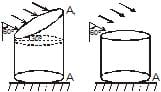Detailed Solution for Test: Fluid Mechanics - 2 - Question 20

In first container the Area vector is along the direction of rain. Rate of water collection in first container is A1​×velocity of rain.
In second cylinder the component of Area vector along the direction of rain is A1​cos60°=A1​/2. So, rate of water collection in second container is 0.5A1​×velocity of rain.
The ratio of water collection is 2.

Test: Fluid Mechanics - 2 - Question 21

large tank is filled with water to a height H. A small hole is made at the base of the tank. It takes T1 time to decrease the height of water to H/h, (h > 1) and it takes T2 time to take out the rest of water. If T1 = T2, then the value of h is :

Detailed Solution for Test: Fluid Mechanics - 2 - Question 21

t=A/a √​2/g​​[√H1​​−√H2​​]
T1​= A​/a√​2/g​​[√H1​​√H​​/η]
T2​=A/a​√​2/g​​[√H/η​−0​]
Given, T1​=T2
√H​−√H​​/η=√H​​/η−0
⇒√H​=2√H​​/η
⇒η=4

Test: Fluid Mechanics - 2 - Question 22

Water flows through a frictionless duct with a cross-section varying as shown in figure. Pressure p at points along the axis is represented by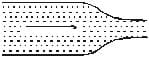Detailed Solution for Test: Fluid Mechanics - 2 - Question 22

When the cross section of  duct decreases the velocity of water increases and in accordance with Bernoulli's theorem the pressure decreases at that place.
Therefore, in this case, the pressure remains constant initially and then decreases as the area of cross section decreases along the neck of the tube and then remains constant along the mouth of the tube.
Hence, the graph in option A is correct.

Test: Fluid Mechanics - 2 - Question 23

A cylindrical vessel filled with water upto the height H becomes empty in time t0 due to a small hole at the bottom of the vessel. If water is filled to a height 4H it will flow out in time

Test: Fluid Mechanics - 2 - Question 24

A tank is filled up to a height 2H with a liquid and is placedon a platform of height H from the ground. The distance x from the ground where a small hole is punched to get the maximum range R is :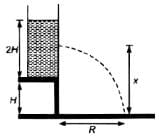Detailed Solution for Test: Fluid Mechanics - 2 - Question 24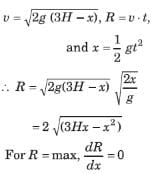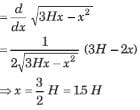Test: Fluid Mechanics - 2 - Question 25

A large tank is filled with water (density = 103 kg/m3). A small hole is made at a depth 10m below water surface. The range of water issuing out of the hole is Ron ground. What extra pressure must be applied on the water surface so that the range becomes 2R (take 1 atm = 105 Pa and g = 10 m/s2) :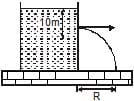Detailed Solution for Test: Fluid Mechanics - 2 - Question 25

Range will become twice, if the velocity of efflux becomes twice. Since velocity of efflux is √2gh​, it will become twice, if h becomes 4 times, or 40m.
Thus, an extra pressure equivalent to 30m of water should be applied.
1atm=0.76×13.6 m of water =10.336m of water
30m of water ≈3atm

## Physics Class 11

127 videos|464 docs|210 tests
 Use Code STAYHOME200 and get INR 200 additional OFF Use Coupon Code
Information about Test: Fluid Mechanics - 2 Page
In this test you can find the Exam questions for Test: Fluid Mechanics - 2 solved & explained in the simplest way possible. Besides giving Questions and answers for Test: Fluid Mechanics - 2, EduRev gives you an ample number of Online tests for practice

## Physics Class 11

127 videos|464 docs|210 tests

### How to Prepare for NEET

Read our guide to prepare for NEET which is created by Toppers & the best Teachers